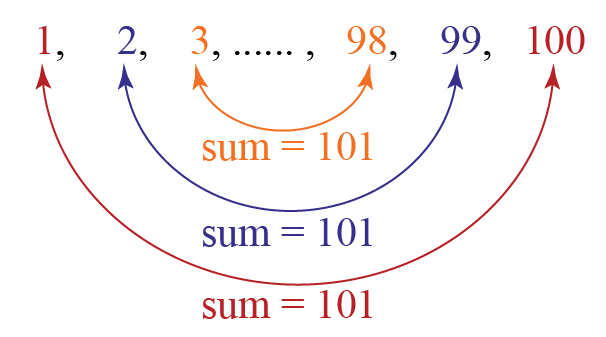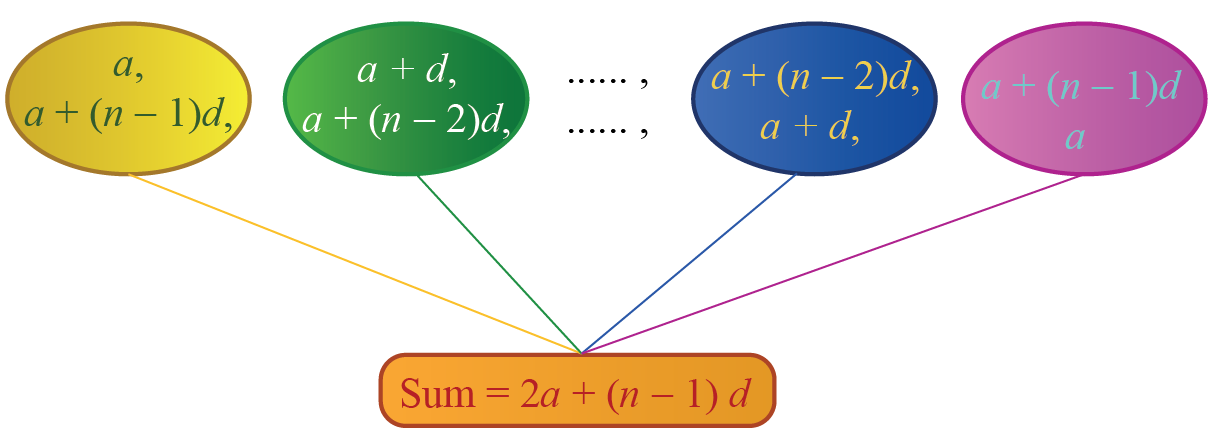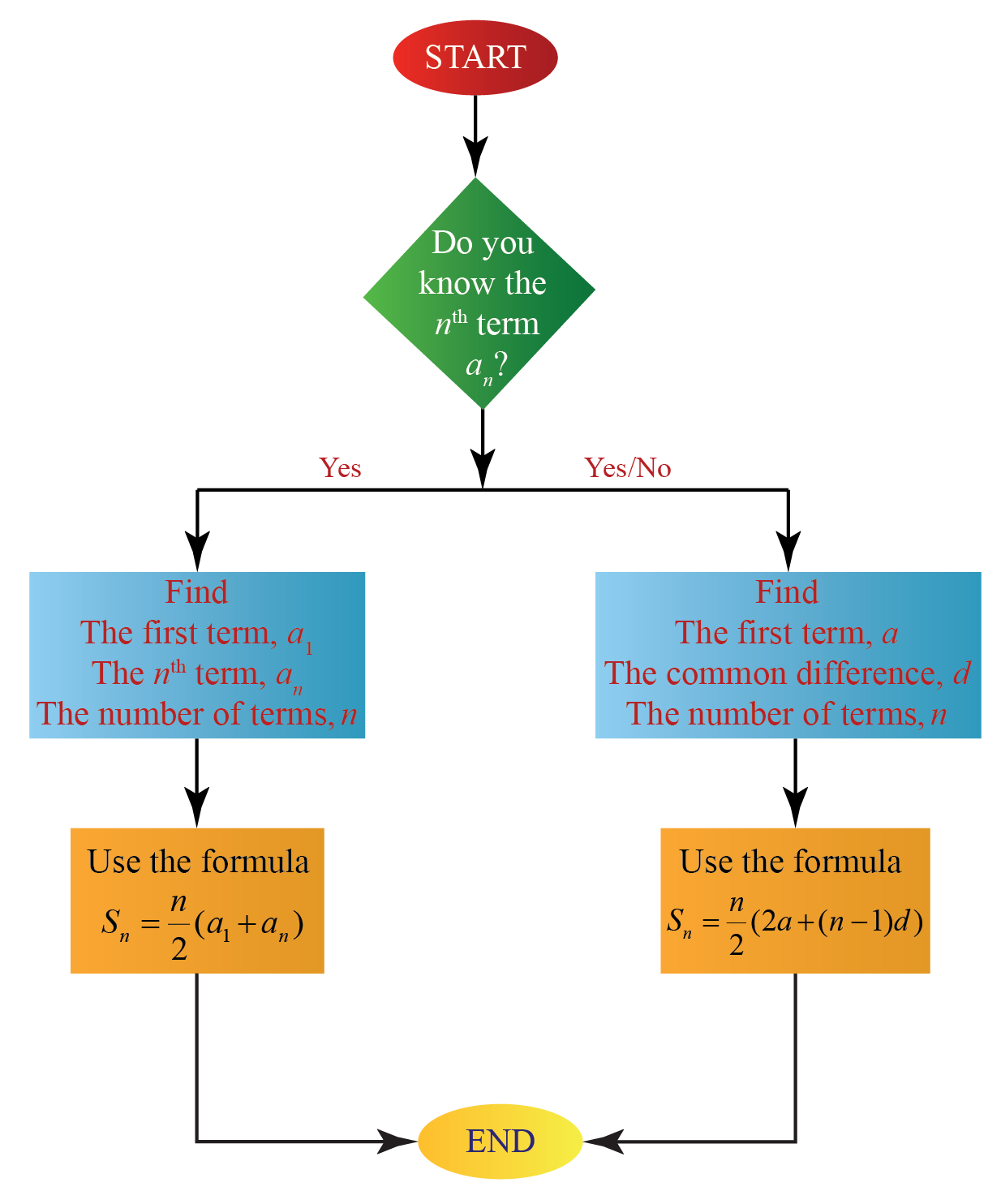# Sum of Arithmetic Sequence

Sum of Arithmetic Sequence

In this mini-lesson, we will explore the sum of an arithmetic sequence formula by solving arithmetic sequence questions. You can also find the sum of arithmetic sequence worksheets at the end of this page for more practice.

In Germany, in the 19th century, a Math class for grade 10 was going on.

The teacher asked her students to add all the numbers from $$1$$ up to $$100$$

The students were struggling to calculate the sum of all these numbers.

One boy shouted out the answer $$5050$$ while the other students were still in the initial steps of calculating the sum.

This boy was the great German mathematician Carl Friedrich Gauss.

How did he arrive at the sum so quickly?

Well, he noticed that terms equidistant from the beginning and the end of the series had a constant sum equal to $$101$$We can see that in the sequence $$1,2,3,...,100$$, there are $$50$$ such pairs whose sum is $$101$$

Thus, the sum of all terms of this sequence is:

$50 \times 101 = 5050$

We use the same logic to find the sum of the arithmetic sequence formula.

## Lesson Plan

 1 How to Find the Sum of an Arithmetic Sequence? 2 Important Notes 3 Solved Examples 4 Challenging Questions 5 Interactive Questions

## How to Find the Sum of an Arithmetic Sequence?

An arithmetic progression is a sequence where the differences between every two consecutive terms are the same.

Examples $\begin{array}{l} 6,13,20,27,34, \ldots \\ 91,81,71,61,51, \ldots \end{array}$

We will see how to derive the sum of arithmetic sequence formula.

Consider an arithmetic sequence  whose first term is $$a_1$$ or $$a$$ and the common difference is $$d$$.

• The sum of the first $$n$$ terms of an arithmetic sequence when the $$n^{th}$$ term is NOT known is:

$S_n=\frac{n}{2}[2 a+(n-1) d]$

• The sum of the first $$n$$ terms of an arithmetic sequence when the $$n^{th}$$ term, $$a_n$$ is known is:

$S_n=\frac{n}{2}[a_1+a_n]$

How do we derive these formulas?

We will use the same logic used above by Carl Friedrich Gauss.

Let us consider the arithmetic sequence with $$n$$ terms:

$a,a+d,a+2d,...(a+(n-2) d),(a+(n-1) d)$

The sum of $$n$$ terms of this sequence is:

$S_n=a+(a+d)+\ldots+(a+(n-2) d)+(a+(n-1) d) \,\,\,\,\,\, \rightarrow (1)$

By reversing the order of the terms of this equation:

$S_n=(a+(n-1) d)+(a+(n-2) d)+\ldots+(a+d)+a \,\,\,\,\,\, \rightarrow (2)$

We see that the sum of corresponding terms of equation (1) and equation (2) yield the same sum which is $$2a+(n-1)d$$.We know that there are totally $$n$$ terms in the above arithmetic sequence.

So by adding (1) and (2), we get:

\begin{align} 2S_n &= n(2a+(n-1) d)\\[0.3cm] S_n &= \frac{n}{2}(2a+(n-1)d) \end{align}

The above sum of arithmetic sequence equation can be written as:

\begin{align} S_n &= \frac{n}{2}(2a+(n-1)d)\\[0.3cm] S_n &= \frac{n}{2}(a+a+(n-1)d)\\[0.3cm] S_n &= \frac{n}{2}(a_1+a_n) [\because a_n=a+(n-1)d \text{ and } a=a_1 ] \end{align}

## Sum of Arithmetic Sequence Formula

From the previous section, the sum of arithmetic sequence equations are:

 \begin{align} S_n &= \frac{n}{2}(2a+(n-1)d)\0.3cm] S_n &= \frac{n}{2}(a_1+a_n) \end{align} Let's take a look at the following flowchart to get an idea of the formula that has to be used to find the sum of arithmetic sequence according to the information available to us.### Sum of Arithmetic Sequence Example Let us find the sum of the first $$n$$ natural numbers using each of the above formulas. The arithmetic sequence of natural numbers is: \[1,2,3,...,n,...

Let us find the sum of $$n$$ terms of an arithmetic sequence of natural numbers.

It would be:

$1+2+3+...+n$

We can find the sum in two methods using the above two formulas.

Method 1

The first term is $$a=1$$

The common difference is $$d=1$$

The number of terms is $$n$$.

Substitute all these values in the first sum of an arithmetic sequence formula:

\begin{align} S_n &= \frac{n}{2}(2a+(n-1)d)\\[0.3cm] S_n &= \frac{n}{2}(2(1)+(n-1)1) \\[0.3cm] S_n&= \frac{n}{2}(2+n-1)\\[0.3cm] S_n&= \frac{n(n+1)}{2} \end{align}

Method 2

The first term is $$a_1=1$$

The nth term of the above arithmetic sequence is $$a_n=n$$.

Substitute all these values in the second sum of an arithmetic sequence formula:

\begin{align} S_n &= \frac{n}{2}(a_1+a_n)\\[0.3cm] S_n&= \frac{n}{2} (1+n)\\[0.3cm] S_n&= \frac{n(n+1)}{2} \end{align}

Thus, from the above methods, the sum of an arithmetic sequence of natural numbers is:

 Sum of an arithmetic sequence of natural numbers $$= \dfrac{n(n+1)}{2}$$

## Sum of Infinite Arithmetic Series

Let us consider an example for the sum of an infinite arithmetic series.

$2+ 5 + 8+...$

Here, the first term is $$a=2$$

The common difference is $$d=3$$

The number of terms is $$n= \infty$$.

Substitute all these values in the formula of sum of an arithmetic series:

\begin{align} S_n &= \frac{n}{2}(2a+(n-1)d)\\[0.3cm] S_n &= \frac{\infty}{2}(2(2)+(\infty-1)3) \\[0.3cm] S_n&= \infty \end{align}

We found the sum of infinite arithmetic series to be $$\infty$$ when

$$d>0$$.

In the same way, the sum of infinite arithmetic series is $$-\infty$$ when

$$d<0$$.

Thus, the sum of infinite arithmetic series is:

## 4. What is the sum of the arithmetic sequence 3, 9, 15..., if there are 34 terms?

Here, the first term is $$a=3$$.

The common difference is $$d=9-3=15-9=...=6$$

The number of terms is $$n=34$$.

The sum is found using the formula:

\begin{align} S_n &= \frac{n}{2}(2a+(n-1)d)\\[0.2cm] &= \frac{34}{2}(2(3)+(34-1)(6))\\[0.2cm] &=3468 \end{align}

More Important Topics
Numbers
Algebra
Geometry
Measurement
Money
Data
Trigonometry
Calculus
More Important Topics
Numbers
Algebra
Geometry
Measurement
Money
Data
Trigonometry
Calculus